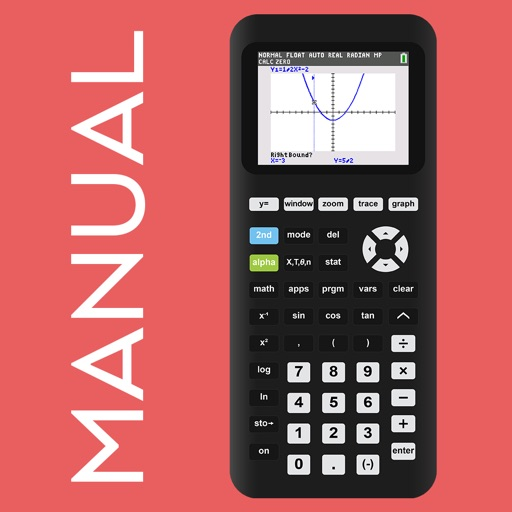## The must-have companion for your TI-84 Plus CE: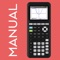# TI-84 CE Calculator Manual

by Graphing Calculator Apps UG (haftungsbeschrankt)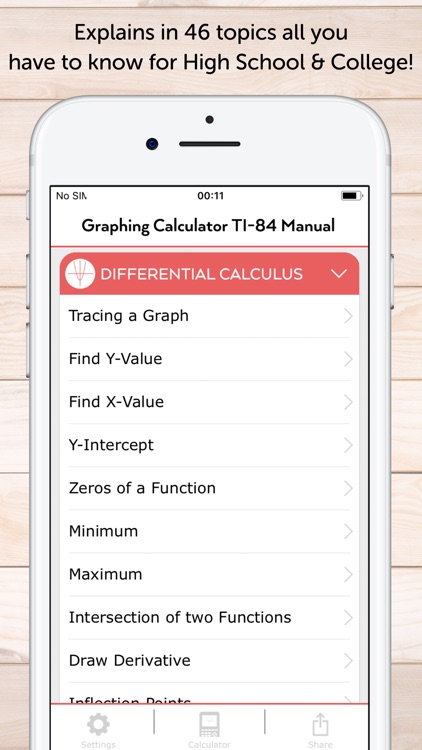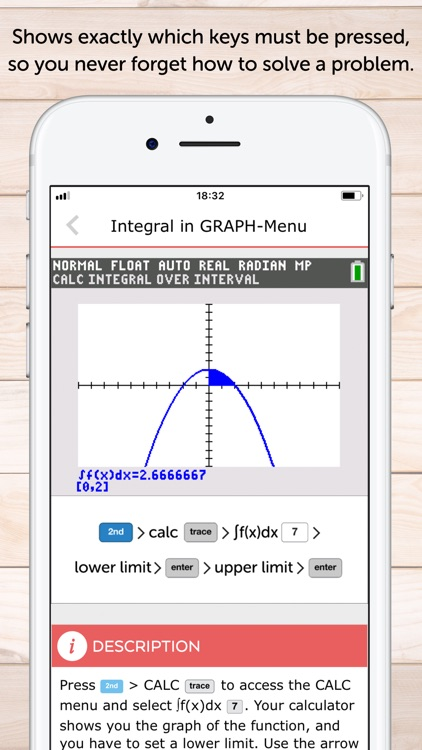The must-have companion for your TI-84 Plus CE:Version
1.5.7
Rating
(78)
Size
41Mb
Genre
Books Education
Last updated
January 4, 2019
Release date
January 9, 2017

### App Screenshots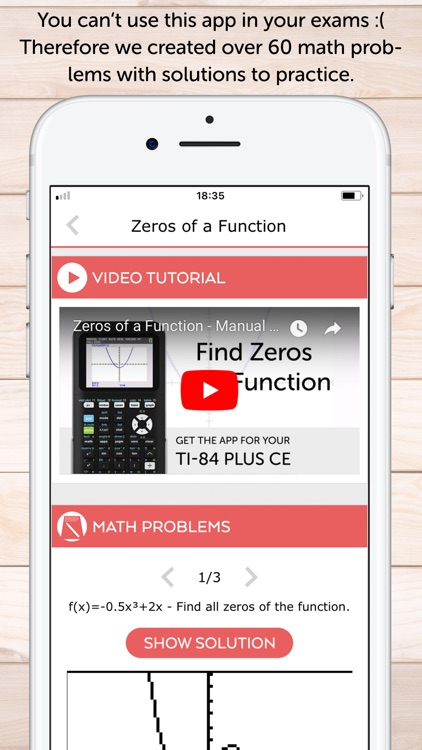### App Store Description

The must-have companion for your TI-84 Plus CE:
• This manual shows all important functions of the graphing calculator TI-84 Plus CE you need to know for high school or college. It comes with step-by-step key press sequences and some secret tips about your calculator. In total it covers 46 topics which will transform you into a TI-84 master.
• The following chapters are available: First Steps, Graphing Basics, Differential Calculus, Solve Equations, Integral Calculus, Matrices and Statistics & Probability.
• You can swipe through screenshots of the original graphing calculator which show every steps you have to do (220 screenshots in total) or watch small animations to see how it works on the TI-84 CE!
• More than 60 math problems with solutions to practice your skills!
• Ideal for exam preparation as you often don't remember all instructions of the calculator after several months. It covers all you need to know for the ACT and SAT.

• The app contains the following topics:

FIRST STEPS
• The Basics of the Basics
• Mode Settings
• Basic Arithmetic
• Storing Variables
• Working with Fractions
• Testing Numbers
• Converting Angles & DMS

GRAPHING BASICS
• Enter Functions
• Enter Families of Functions
• Formatting the Graph
• Setting the Graph Window
• Zooming the Graph Window

DIFFERENTIAL CALCULUS
• Tracing a Graph
• Find Y-Value
• Find X-Value
• Y-Intercept
• Zeros of a Function
• Minimum
• Maximum
• Intersection of two Functions
• Draw Derivative
• Inflection Points
• Calculate Tangent

SOLVE EQUATIONS
• Polynomial
• Solve any Equation
• Equation Solver

INTEGRAL CALCULUS
• Calculate Integral
• Find Area with Absolute Value
• Area between two Functions
• Integral Function

MATRICES
• Save Matrix
• Delete Matrix
• Put into Row Echelon Form
• Solve Matrix
• Determinant of a Matrix
• Transpose of a Matrix
• Identity Matrix
• Inversion of a Matrix
• Matrix Arithmetic Operations

STATISTICS & PROBABILITY
• Permutations, Combinations & Factorials
• Random Numbers
• Lists & Statistical Data
• Histogram, Box Plot & Scatter Plot
• Statistical Data Analysis
• Regression

MATH PROBLEMS
• Practice with over 60 math problems and solutions.

We also have apps for the calculators: TI-Nspire CX (and CAS), TI-84 Plus, TI-84 Plus CE, CASIO fx-9860GlI

NO ACTUAL GRAPHING CALCULATOR INCLUDED. THE APP IS A MANUAL!

Disclaimer:
AppAdvice does not own this application and only provides images and links contained in the iTunes Search API, to help our users find the best apps to download. If you are the developer of this app and would like your information removed, please send a request to [email protected] and your information will be removed.